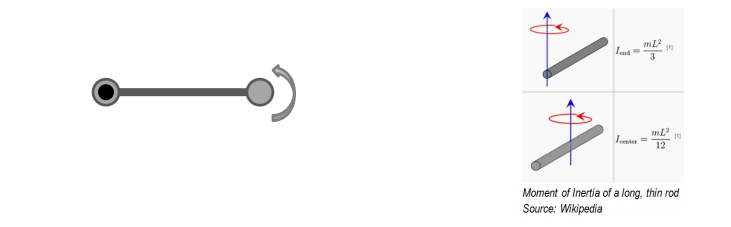Start typing, then use the up and down arrows to select an option from the list.## Physics

Learn the toughest concepts covered in Physics with step-by-step video tutorials and practice problems by world-class tutors

16. Angular Momentum

# Angular Momentum of a Point Mass

1
concept

## Angular Momentum of a Point Mass8m
Play a video:
2
Problem

The Earth has mass 5.97 × 1024 kg, radius 6.37 × 106 m. The Earth-Sun distance is 1.5 × 1011 m. Calculate its angular momentum as it spins around itself. Treat the Earth as a solid sphere of uniform mass distribution.

BONUS 1:Treating the Earth as a point mass, calculate its angular momentum as it spins around the Sun.

BONUS 2:Does the Earth have linear momentum as it spins around (i) itself; (ii) the Sun?

3
Problem

A system is made of two small, 3 kg masses attached to the ends of a 5 kg, 4 m long, thin rod. The system rotates with 180 RPM about an axis perpendicular to the rod and through one of its ends, as shown. Calculate the system’s angular momentum about its axis.